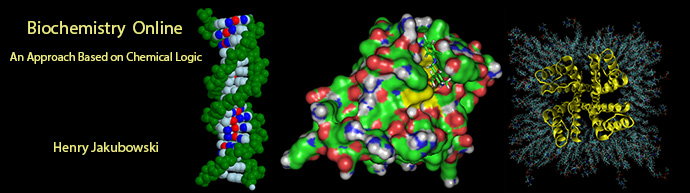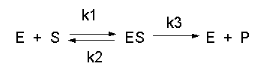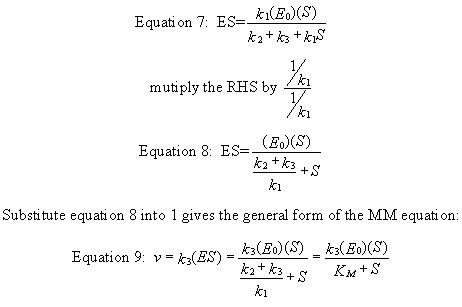# Biochemistry Online: An Approach Based on Chemical Logic# CHAPTER 6 - TRANSPORT AND KINETICS

## B:  Kinetics of Simple and Enzyme-Catalyzed Reactions

BIOCHEMISTRY - DR. JAKUBOWSKI

4/10/16

 Learning Goals/Objectives for Chapter 6B:  After class and this reading, students will be able to write appropriate chemical and differential equations for the rate of disappearance of reactants or appearance of products for 1st order, pseudo first order, second order, reversible first order reactions draw and interpret graphs for integrated rate equations (showing reactant or product concentrations as a function of time) and initial rate equations (showing the initial velocity vo as a function of reactant ; derive kinetic rate constants from data and graphs of integrated rate and initial rate equations; write appropriate chemical, differential equations, and initial rate equations for the rate of disappearance of reactants or appearance of products for simple enzyme catalyzed reaction; differentiate between rapid and steady state assumptions; simplify the initial rate equation containing rate constants for an enzyme catalyzed reactions to one replacing the rate constants with kcat and KM, and give operational and mathematical definitions of those constants;

# B4.  Steady State Enzyme-Catalyzed ReactionsIn this derivation, we will consider the following equations and all the rate constants, and will not arbitrarily assume that k2 >> k3. We  will still assume that S >> Eo and that Po = 0. An added assumption, however, is that dES/dt is approximately 0. Look at this assumption this way. When an excess of S is added to E, ES is formed. In the rapid equilibrium assumption, we assumed that it would fall back to E + S faster than it would go onto product. In this case, we will assume that it might go on to product either less or more quickly than it will fall back to E + S. In either case, a steady state concentration of ES arises within a few milliseconds, whose concentration does not change significantly during the initial part of the reaction under which the initial rates are measured. Therefore, dES/dt is about 0. In the rapid equilibrium derivation, we observed that v = k3ES.   We then solved for ES using Ks and mass balance of E. In the steady state assumption, the equation v = k3ES still holds, but know we will solve for ES using the steady state assumption that dES/dt =0.

Equation 1)    v = k3ES

Equation 6: (steady state) dES/dt = k1(E)(S) - k2(ES) -k3(ES) = 0

k1(E)(S) = (k2 + k3)(ES)

k1(Eo-ES)(S) = (k2 + k3)(ES)

k1(Eo)(S) - k1(ES)(S) = (k2 + k3)(ES)

k1(Eo)(S) = (k2 + k3)(ES) + k1(ES)(S)

k1(Eo)(S) = ES (k2 + k3 + k1S)Equation 9)   v = k3ES = [k3(Eo)(S)]/ [(k2 + k3)/k1 + S] = [k3(Eo)(S)]/(Km+ S)Navigation

Archived version of full Chapter 5B:  Kinetics of Simple and Enzyme-Catalyzed Reactions